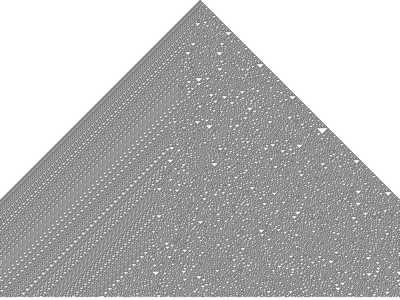# Cellular Automata

Simple cellular autamata engine, designed with Python+Pygame.(vkataev)
Simple cellular autamata engine, designed with Python+Pygame. A cellular automaton (plural: cellular automata) is a discrete model studied in computability theory, mathematics, and theoretical biology. It consists of a regular grid of cells, each in one of a finite number of states. The grid can be in any finite number of dimensions. Time is also discrete, and the state of a cell at time t is a function of the states of a finite number of cells (called its neighborhood) at time t − 1. These neighbors are a selection of cells relative to the specified cell, and do not change (though the cell itself may be in its neighborhood, it is not usually considered a neighbor). Every cell has the same rule for updating, based on the values in this neighbourhood. Each time the rules are applied to the whole grid a new generation is created.

### Changes